Power, intensity and decibels

Consider a wave on a string. Any point along the string moves up and down as a wave passes through it. The average velocity of a point on the string is related to the amplitude of the wave and to how quickly the motion changes from up to down – thus the frequency.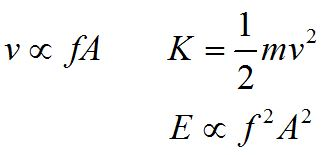The kinetic energy of the mass at that point depends on the square of the velocity. We can infer that the energy of the wave goes as the square of the amplitude, and the square of the frequency.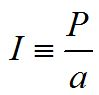We define the intensity I of a wave to be the power delivered by the wave, divided by the area that the wave impinges upon. The units of intensity are Watts per square meter.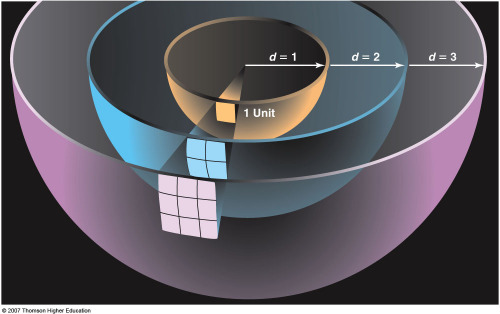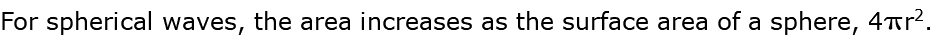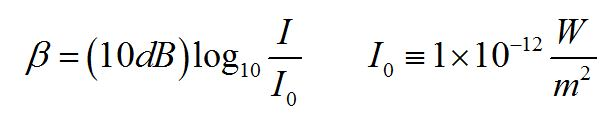When I = I0, the sound intensity level is zero decibels. This doesn't mean that there are no sound waves present, just that humans cannot hear them.

We start to feel pain from high intensity sound level at about 130 dB.

Practice problems

1. If the amplitude of a sound wave doubles, how much does the intensity increase? What is I2/I1?

2. You are standing 12 m from a speaker and measure the intensity of the sound to be 240 W/m2. Then you move to 24 m from the speaker. What intensity do you measure?

3. The intensity level of sound waves for normal conversation is about 60 dB.

What is the intensity of sound at 60 dB?

4. What is the intensity level if two people are talking?